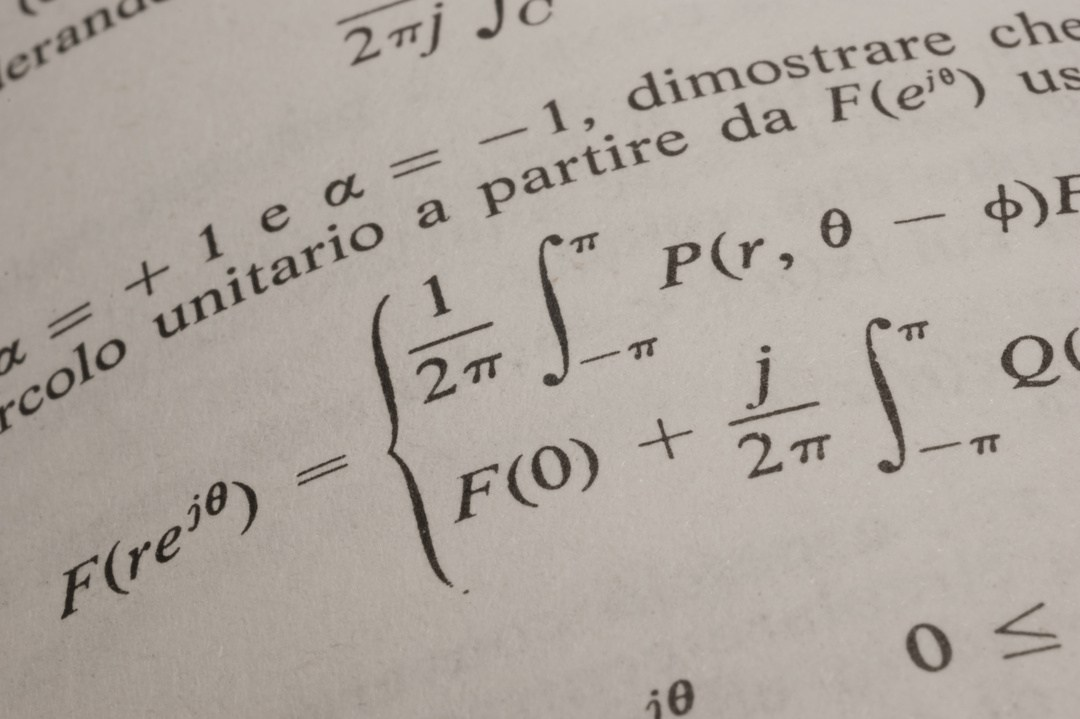# Factoring Algebra Expressions with Variables

Students studying algebra in Tustin can often benefit from the help of a home algebra tutor when it comes to factoring. Knowing the common algebra factors of numbers and algebra variables is the first step in factoring. From there,Students studying algebra in Tustin can often benefit from the help of a home algebra tutor when it comes to factoring.  Knowing the common algebra factors of numbers and algebra variables is the first step in factoring.  From there, a home algebra tutor can teach his/her Tustin student about the FOIL method of multiplying and factoring algebra expressions.

To review, let’s start with a couple of basic algebra factoring examples:

• Factor 6x⁴ + 12x⁵
• First off, what algebra factors do these two quantities have in common?
• Both can be divided by 1, 2, 3, and 6.  As 6 is the largest number in common, it is the best factor to use.
• Both also have “x” raised to an exponent.  What is the greatest number of x’s that can be divided out of both quantities?  You can divide out four x’s, therefore divide by x⁴.
• 6x⁴/6x⁴ = 1 and 12x⁵/6x⁴ = 2x
• The final algebra answer is 6x⁴(1 + 2x) or, written in standard form, 6x⁴(2x + 1)

The reverse of this question could be: Multiply 6x⁴(2x + 1).  As we learned in the first algebra example, the answer would be 12x⁵ + 6x⁴.  But what about when there are multiple quantities to be multiplied in basic algebra?

How can a Tustin algebra student solve (2x + 1)(4x + 5)?

In this case, your home algebra tutor can explain the F.O.I.L. method of multiplying.

In basic algebra, this stands for First, Outer, Inner, Last, and is an easy way to remember which basic algebraic quantities to multiply.

• Multiply (2x +1)(4x +5)
• Using FOIL, the steps are:
• First: 2x x 4x = 8x 2
• Outer: 2x x 5 = 10x
• Inner: 1 x 4x = 4x
• Last:  1 x 5 = 5
• Combining these, we find: 8x 2  + 10x + 4x + 5
• Combining like terms results in the answer: 8x 2  + 14x + 5

With enough practice and the help of a home algebra tutor, a Tustin algebra student will have the FOIL method memorized in no time!

Get yourself on the right track by hiring an excellent home algebra tutor to come on a weekly or biweekly basis at the start of the school year to learn more on basic algebra factoring alongside algebra variables.

A Plus In Home Tutors offers tutoring for all subjects including basic algebra, such as basic algebra factoring, in Tustin, Orange, Irvine, and throughout Orange County.

An algebra tutor in Tustin can help increase your comprehension and your test scores.

Earn an “A” in Algebra this year!  Visit  www.FireflyTutors.com.